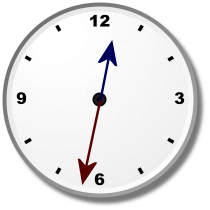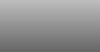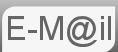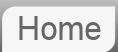# Answer to Puzzle #35: When the Hands of a Clock First Overlap

35. A regular clock has an hour and minute hand. At 12 midnight the hands are exactly aligned. When is the next time they will exactly align or overlap? How many times a day will they overlap?

I thought of this puzzle as a way of explaining another puzzle. But it's quite a good one so we can cover it itself...Before reading the answer can I interest you in a clue?

(The 'Hands in a Straight Line' problem is further down the page.) First thing is the answer is not 1:05 which might be your first thought. Why? As we dealt with in puzzle #5 by the time the minute hand gets to five past one the hour hand will have moved slightly past 1 o'clock. The hour hand after all does not wait at 1 o'clock for an hour but rather it moves to 2 o'clock over the course of the next hour. The first overlap will be a little after five past one.

The first approach is probably the most obvious; it's the most mechanically intuitive. We'll use a variable 't' the time in hours. We know the speeds of the hands by definition the Minute Hand will move one full rotation or 360° per hour. The Hour Hand makes a full rotation in 12 hours and will therefore move at 30° per hour. At our first overlap just after five past one the Minute Hand will have done one full rotation plus the bit we are interested in. The Hour Hand will have done just a part rotation of 't' times it's speed.
```Hour Hand = Minute Hand
30t = 360t - 360
t = 12(t - 1)
11t = 12
t = 12/11
= 1.090909.. hours
= 65.454545454.. minutes
= 1h 5min 27.272727..seconds
```
The next method i would like to suggest is that we realise that the concept of 360 degrees is an artificial construction. And not necessary to solve the problem. We can consider the speeds only in term of revolutions per hour with the Minute and Hour Hands moving at 1 and 1/12 revolutions per hour respectively. The first line of our equation then becomes:
```Hour Hand = Minute Hand
t/12 = t - 1
```
Probably the most elegant solution is to realise that the overlap will happen 11 times in a 12 hour period and so the answer is just 1/11th of 12 hours. It's difficult to divine this. But it makes sense. Just as we reasoned our first overlap will be at just after 1:05 we can reason that our last will be just before 10:55. Our overlaps will be at just after 1:05, a bit more after 2:10, even more after 3:15 and so on (actual times give at the end) but since our last is a bit before 10:55 there will be no overlap whilst the Hour Hand is indicating 11. A Very Scientific Approach below also gives us a way to realise this.

I found the whole problem a lot easier to visualise by playing with the flash based clock below. You can drag the hands with your mouse.

## A Very Scientific Approach

The final method is very physics. I want to look at the concept of reference frames, the idea has many applications in physics, it's essential for example in general relativity or when we consider something like how fast is the light traveling away from the head lights of a fast moving car. But it also has some much more mundane applications. Simply we redefine our co-ordinate system so that it's stationary with respect to one of our bodies. An example of this would be if we have two cars moving at different speeds, lets say 30 and 35mph. The faster car is a mile behind, when will it overtake? We can solve the equation of motion 30t+1=35t, but what we might do intuitively is move to the frame of reference of the lead car. In this reference frame the lead car is stationary and the car behind is a mile away approaching at 5mph. That's essentially a one dimensional problem and a bit too easy to solve. But if you are considering say the intercept courses of ships in two dimensions it becomes more useful.

We will make our reference frame that of the Hour Hand. (Either would work.) The problem will exist from the perspective of someone stood on the Hour Hand with no other visual cues; all he can see is the minute hand. In the external reference frame the Minute Hand moved with speed (or angular velocity, since we are now speaking physics) 1 revolutions per hour and the Hour Hand with 1/12th. To move into the frame of reference of the Hour Hand we subtract it's speed from that of the Minute Hand to give the Minute Hand's speed in the Hour Hand's reference frame. As in 1 - 1/12th. In our new frame of reference the Minute Hand moves at an angular velocity of 11/12ths revolutions per hour. Coincidence or overlap occurs every time the Minute Hand makes a complete revolution. The question of how many revolutions something moving at 11/12ths revolutions per hour makes in a 12 hour period is trivial.

If we have by now proven that there are 11 overlaps in a 12 hour period, clearly there are 22 overlaps in a 24 hour period.

## Hands form a straight lineThis comes up from time to time. The method is basically the same as above but lets go for it.

The first approach is probably the most obvious; it's the most mechanically intuitive. We'll use a variable 't' the time in hours. We know the speeds of the hands by definition the Minute Hand will move one full rotation or 360° per hour. The Hour Hand makes a full rotation in 12 hours and will therefore move at 30° per hour. The first straight line after midnight will be just after half an hour. We are looking for a point where the position of the hour hand plus 180 degrees is equal to the position of the minute hand.
```Hour Hand + 180 = Minute Hand
30t + 180 = 360t
t + 6 = 12t
6 = 11t
t = 6/11
= .545454.. hours
= 32.727272.. minutes
= 32min 43.636363..seconds
```

## Full List of Overlap Times

Both AM and PM
01:05:27
02:10:55
03:16:22
04:21:49
05:27:16
06:32:44
07:38:11
08:43:38
09:49:05
10:54:33
12:00:00

© Nigel Coldwell 2004 -  – The questions on this site may be reproduced without further permission, I do not claim copyright over them. The answers are mine and may not be reproduced without my expressed prior consent. Please inquire using the link at the top of the page. Secure version of this page.

PayPal
I always think it's arrogant to add a donate button, but it has been requested. If I help you get a job though, you could buy me a pint! - nigel